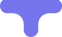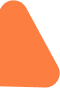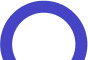### Master Math with 1:1 Online Classes. Learn from Experts!# Numbers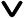• Numerals and Digits
• Numeration Systems
• Roman Numerals
• History of Numbers
• Counting Numbers
• Number Names
• Number Line
• Comparing and Ordering Numbers
• Odd and Even Numbers
• Prime Numbers
• Composite Numbers
• Ordinal Numbers
• Types of Numbers
• Natural Numbers
• Whole Numbers
• Integers
• Fractions
• Rational Numbers
• Irrational Numbers
• Real Numbers
• Complex Numbers
• Solved Examples
• Practice Problems

Numbers are more than just objects or quantities that we can see and touch. They are ideas that go beyond the physical world. They represent quantities and magnitudes and serve as a fundamental part of mathematics. Take the number "3" as an example. It can represent three apples, three kilometers, or even three minutes. Regardless of what it represents, the number "3" holds a consistent value. This consistency allows us to compare different quantities, perform calculations, and establish relationships between numbers.Numbers provide a universal language for communication and analysis, allowing us to make comparisons, perform calculations, and establish relationships across various contexts.

### Related Topics

 Natural Numbers Whole Numbers Prime Numbers Roman Numerals Integers Fractions Decimal Numbers Rational Numbers Complex Numbers Fibonacci Numbers Irrational Numbers Real Numbers

## Digits and Numerals

Imagine numerals as special signs that represent different numbers. Just like how we have letters to write words, we use numerals to express numbers. For example, the numeral "7" represents the number seven. So, numerals are like codes that tell us the value of the number.Now, let's talk about digits. Digits are like tiny puzzle pieces that help create numerals. We have ten special symbols called digits: 0, 1, 2, 3, 4, 5, 6, 7, 8, and 9. These digits are the building blocks of numerals.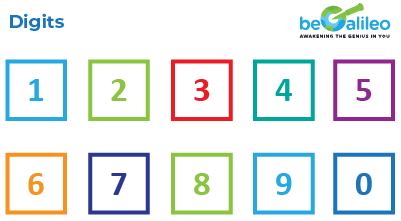For instance, the numeral 64 is formed by combining two digits: "6" and "4." Even a single digit, like "2," can be a numeral by itself, representing the number two.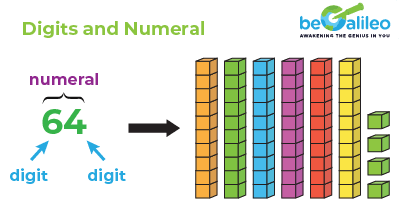So, think of numerals as secret codes for numbers, and digits as the unique puzzle pieces used to create these codes. Understanding numerals and digits allows us to read and write numbers easily, which is important for various activities in our everyday life and mathematics.

## Numeration Systems

In mathematics, we use various writing systems, known as numeration systems, to represent numbers. The one we commonly encounter employs digits from 0 to 9, and it is called the Hindu-Arabic system. This system allows us to write all kinds of numbers using these digits.

Additionally, you might have come across Roman Numerals like VI and X, which are part of the Roman Numeration System. Roman Numerals have a different set of symbols and rules for expressing numbers.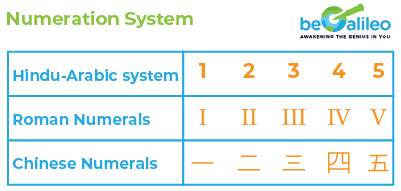Each numeration system has its unique way of representing numbers, making math fascinating and diverse.

## History of Numbers

The history of numbers dates back thousands of years and is closely intertwined with the development of human civilization. Numbers have played a crucial role in various aspects of human life, including trade, agriculture, architecture, astronomy, and more. Let's take a brief journey through the history of numbers

The earliest evidence of numerical systems can be traced back to ancient civilizations such as the Sumerians, Egyptians, and Indus Valley Civilization. These civilizations developed their own numeral systems to represent quantities and perform calculations.

The Sumerians used a base-60 system, which led to the creation of the concept of minutes and seconds in measuring time. The Egyptians employed a decimal system, using hieroglyphs to represent numbers.One of the most significant developments in the history of numbers came from the ancient Indian mathematicians. Around the 5th century BCE, the Indians introduced the concept of zero and the decimal place-value system. This revolutionary idea of positional notation made arithmetic operations more efficient and laid the foundation for modern mathematics. It eventually spread to other parts of the world, including the Arab world.

During the Islamic Golden Age, scholars such as Al-Khwarizmi made significant contributions to mathematics. Al-Khwarizmi's book "Kitab al-Jabr wa al-Muqabala" introduced algebra and algorithms, which further advanced the understanding and use of numbers.

In Europe, the adoption of Arabic numerals, which included the digit zero, gained prominence during the Middle Ages. It revolutionized mathematical computations and made complex calculations more accessible. The Italian mathematician Fibonacci introduced the Hindu-Arabic numeral system to Europe in the 13th century, further popularizing its use.

The Renaissance period witnessed significant advancements in mathematics, with notable mathematicians like Leonardo da Vinci and Galileo Galilei contributing to the understanding of numbers and their applications. The Scientific Revolution of the 17th century, led by scientists such as Sir Isaac Newton and Gottfried Wilhelm Leibniz, brought about further developments in mathematics, including the invention of Calculus.

In modern times, the field of mathematics has witnessed remarkable progress, with the exploration of complex numbers, set theory, number theory, and the discovery of irrational and transcendental numbers. These developments have greatly expanded our understanding of numbers and their relationships.

## Counting Numbers

Counting Numbers also known as natural numbers, are the numbers we use to count or enumerate objects. They start from 1 and continue indefinitely. The set of counting numbers is denoted as N = {1, 2, 3, 4, 5, ...}.

Example: Counting numbers from 1 to 10 are 1, 2, 3, 4, 5, 6, 7, 8, 9, 10.## Number Names

Number names are the words used to represent numbers in written form. Each number has a specific word associated with it. For example, the number 5 is represented as "five" in words. Number names are important for reading and writing numbers and understanding their place value.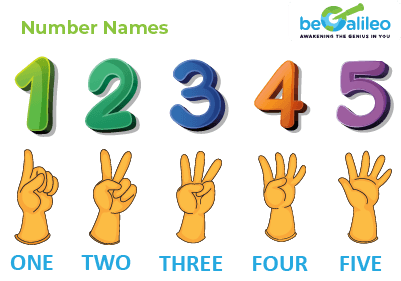Example: The number 35 is written as "thirty-five" in number names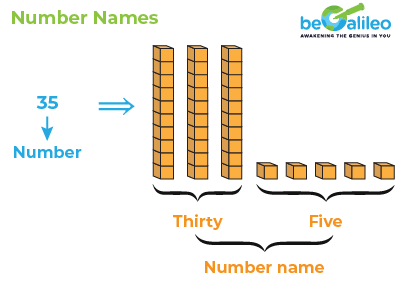## Number Line

A number line is a visual representation of numbers arranged in order on a straight line. It helps us understand the relative positions and magnitudes of different numbers. The number line extends infinitely in both directions, with zero at the center. Positive numbers are represented to the right of zero, while negative numbers are represented to the left.

Example: Consider a number line with the range -5 to 5. The numbers are positioned as follows:

-5 -4 -3 -2 -1 0 1 2 3 4 5## Comparing and Ordering Numbers

Comparing and ordering numbers is the process of arranging numbers in increasing or decreasing order based on their values. It helps us understand the relative sizes and magnitudes of different numbers. For example, when comparing the numbers 3 and 7, we can determine that 7 is greater than 3.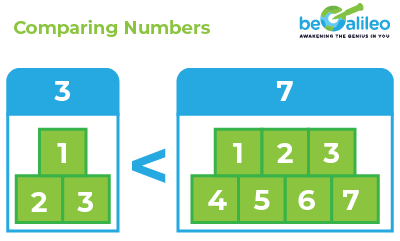Example: Order the numbers 8, 3, 12, and 5 in ascending order: 3, 5, 8, 12.## Odd and Even Numbers

Numbers can be classified as odd or even based on their divisibility by 2. Even numbers are divisible by 2, while odd numbers are not. For example, 4 and 10 are even numbers, while 5 and 11 are odd numbers. Understanding odd and even numbers helps in various mathematical operations and patterns.

Example: Identify whether the numbers 7, 12, 15, and 20 are odd or even: 7 (odd), 12 (even), 15 (odd), 20 (even).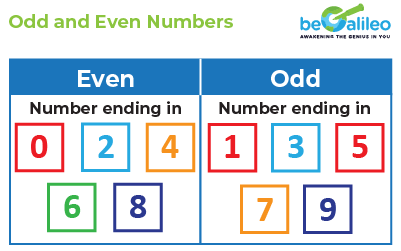## Prime Numbers

Prime numbers are numbers greater than 1 that have exactly two factors: 1 and the number itself. In other words, they are not divisible by any other number except 1 and themselves. For example, 2, 3, 5, 7, 11, and 13 are prime numbers. Prime numbers have fascinating properties and play a crucial role in number theory and cryptography.

Example: Identify the prime numbers between 1 and 20: 2, 3, 5, 7, 11, 13, 17, 19.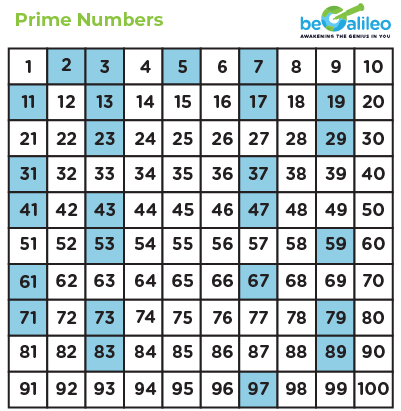## Composite Numbers

Composite numbers are numbers that have more than two factors. In other words, they are divisible by numbers other than 1 and themselves. For example, 4, 6, 8, 9, and 10 are composite numbers. Understanding composite numbers helps in identifying patterns and relationships between numbers.

Example: Identify the composite numbers between 1 and 20: 4, 6, 8, 9, 10, 12, 14, 15, 16, 18, 20.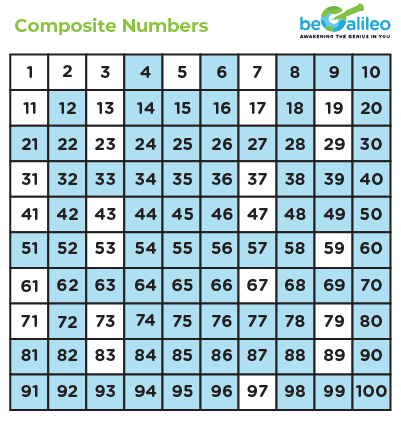## Ordinal Numbers

Ordinal numbers represent the position or order of objects in a sequence. They are derived from counting numbers and indicate the rank or place of an object in a series. For example, first, second, third, and so on. Ordinal numbers are important for understanding rankings, ordering, and arranging objects in a specific order.Example: Write the ordinal numbers for the counting numbers 1 to 5: first, second, third, fourth, fifth## Types of Numbers

Numbers can be categorized into different types based on their properties. The numbers are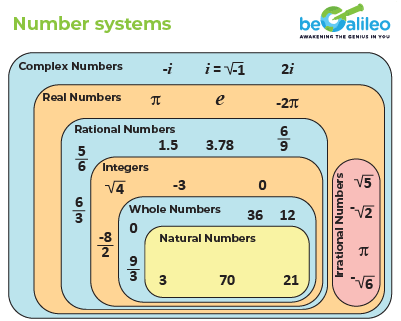### Natural Numbers

Natural numbers are the counting numbers that start from 1 and continue indefinitely. They are denoted by N = {1, 2, 3, 4, 5, ...}.

Example: 1, 2, 3, 4, 5, ...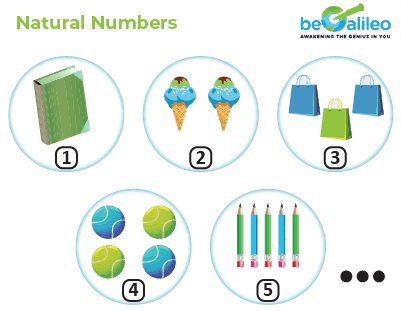### Whole Numbers

Whole numbers include natural numbers along with zero. They are denoted by W = {0, 1, 2, 3, 4, ...}.

Example: 0, 1, 2, 3, 4, ...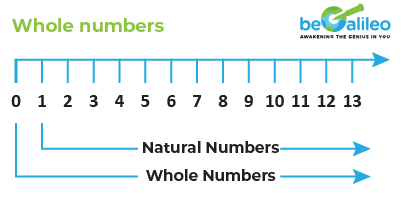### Integers

Integers are numbers that include both positive and negative whole numbers along with zero. They are denoted by Z = {..., -3, -2, -1, 0, 1, 2, 3, ...}.

Example: ..., -3, -2, -1, 0, 1, 2, 3, ...### Fractions

Fractions represent a part of a whole or a ratio between two numbers. They are expressed as \frac{a}{b}, where a is the numerator and b is the denominator. For example,\frac{1}{2} ,\frac{3}{4} and \frac{5}{6} are fractions.

Example: \frac{1}{2} ,\frac{3}{4},\frac{5}{6}, ...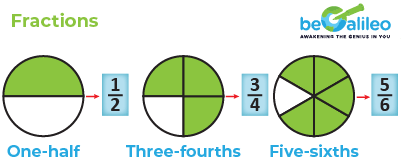### Rational Numbers

Rational numbers include integers and fractions. They can be expressed as the ratio of two integers. Rational numbers can be positive, negative, or zero. For example, \frac{1}{3} ,\frac{-5}{2}, and 4 are rational numbers.

Example: \frac{1}{3} ,\frac{-5}{2}, 4, ...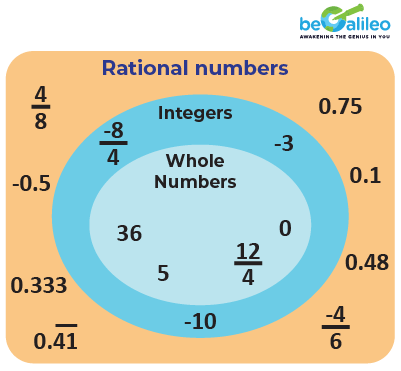### Irrational Numbers

Irrational numbers cannot be expressed as fractions or ratios. They are non-repeating and non-terminating decimals. Examples of irrational numbers include \sqrt{2}, π (pi), and e (Euler's number).

Example:\sqrt{2}, π, e, ...

### Real Numbers

Real numbers include both rational and irrational numbers. They represent all possible numbers found in the real world. Real numbers can be positive, negative, or zero. They are denoted by the symbol R

Example: -∞, ..., -3, -2, -1, 0, 1, 2, 3, ..., ∞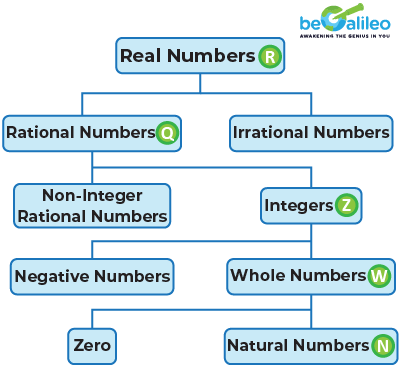### Complex Numbers

Complex numbers consist of a real part and an imaginary part. They are represented in the form a + bi, where a and b are real numbers, and i is the imaginary unit (\sqrt{-1}). Complex numbers are used in advanced mathematics and have applications in fields like engineering and physics.

Example: 2 + 3i, -4 + 5i, ...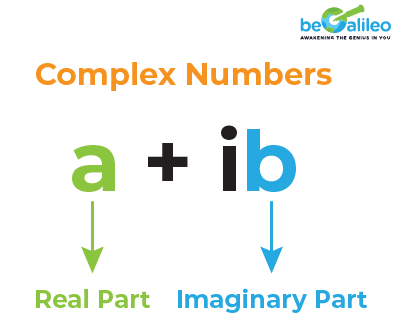## MATH WORKSHEETS

• The number zero (0) is neither positive nor negative and is considered an important mathematical concept.
• The number 1 is unique as it is not classified as a prime number or a composite number.
• The number pi (π) is an irrational number that represents the ratio of a circle's circumference to its diameter. It is approximately equal to 3.14159 and is used in various mathematical calculations.
• The Fibonacci sequence is a series of numbers in which each number is the sum of the two preceding ones. It has intriguing mathematical properties and can be found in various natural phenomena. For example: 1, 1, 2, 3, 5, 8 ....
• The number 7 is often considered lucky in many cultures, while the number 13 is associated with superstitions and considered unlucky in some traditions

## Solved Examples

### Q1. Which of the following numbers is an integer?

1. a) 2\sqrt{7}
2. b) 2\sqrt{4}
3. c) 0\cdot90
4. d) \sqrt{6}

Solution: The correct answer is 2\sqrt{4}.
As \sqrt{4} = 2, 2\times 2 = 4.

### Q2. Identify the rational number.

1. a) 2\sqrt{2}
2. b) \pi
3. c) 0.\overline{3}
4. d) -\sqrt{5}

Solution: The correct answer is 0.\overline{3}.
0.\overline{3} is the only rational number as the recurring decimal can be written as a fraction, \frac{1}{3}

### Q3. Identify the natural numbers among the given numbers:

1. a) 0
2. b) 23
3. c) 98
4. d) \frac{11}{7}
5. f) 3
6. g) -98

Solution: Since negative numbers, 0, decimals, and fractions are not a part of natural numbers. Therefore, 0, -98, and \frac{11}{7}are not natural numbers. Therefore, natural numbers are 23, 98, and 3.

### Q4. Are all the fractions rational numbers?

1. a) Yes
2. b) No

Solution: Fractions are numbers that are represented in the form of (numerator/denominator), which is equivalent to \frac{p}{q} form, so fractions are considered rational numbers.

Example: \frac{2}{5} is a fraction and it is also a rational number.

### Q5. Are all rational numbers fractions?

1. a) Yes
2. b) No

Solution: All fractions are rational numbers, but all rational numbers are not fractions

Example: \frac{12}{17} is a fraction and a rational number whereas -\frac{12}{17} is a rational number and not a fraction.

## FAQs - Understanding Numbers

Digits are the individual symbols used to create numerals. In our everyday numerals, we use ten digits: 0, 1, 2, 3, 4, 5, 6, 7, 8, and 9. Numerals, on the other hand, are combinations of these digits used to represent numbers. For example, the numeral "153" is made up of three digits: "1", "5", and "3".

Numeration systems are different methods used to represent numbers in written form. The most familiar system uses digits 0 to 9, known as the Hindu-Arabic system. However, there are other systems like Roman Numerals, which use a distinct set of symbols to represent numbers.

Roman Numerals are a special numeration system that uses symbols like I, V, X, L, C, D, and M to represent numbers. Unlike the Hindu-Arabic system, Roman Numerals have specific rules for combining symbols to form numerals.

The history of numbers traces the development of numerical systems and their usage throughout different civilizations and time periods. It includes the evolution of various numeration systems and their contributions to modern mathematics

Counting numbers are the natural numbers that start from 1 and continue indefinitely. They are used for counting objects or items in a set. For example, 1, 2, 3, 4, 5, and so on are counting numbers.

Number names are the words used to represent numerical values. For instance, "twelve" is the number name for the numeral 12.

The number line is a visual representation of numbers arranged in increasing or decreasing order. It helps us understand the order and relative magnitude of numbers.

Comparing numbers involves determining whether one number is greater than, less than, or equal to another. Ordering numbers involves arranging them in ascending or descending order.

Odd numbers are numbers that are not divisible by 2 and leave a remainder of 1 when divided by 2. Even numbers, on the other hand, are exactly divisible by 2, leaving no remainder.

Prime numbers are natural numbers greater than 1 that have only two factors: 1 and the number itself. For example, 2, 3, 5, 7, and 11 are prime numbers.

Composite numbers are natural numbers greater than 1 that have more than two factors. In other words, they can be divided by more than just 1 and themselves.

Ordinal numbers represent the position or order of objects in a sequence. For example, 1st, 2nd, 3rd, 4th, and so on are ordinal numbers.

Natural numbers are the counting numbers that start from 1 and continue indefinitely. They are represented as N = {1, 2, 3, 4, ...}.

Whole numbers are the set of natural numbers along with zero. They are represented as W = {0, 1, 2, 3, ...}.

Integers are the set of positive whole numbers, negative whole numbers, and zero. They are represented as Z = {..., -3, -2, -1, 0, 1, 2, 3, ...}.

Fractions are numbers that represent a part of a whole. They are written in the form \frac{a}{b}, where "a" is the numerator and "b" is the denominator.

Rational numbers are numbers that can be expressed as fractions. They can be written in the form \frac{p}{q}, where "p" and "q" are integers and "q" is not equal to zero.

Irrational numbers cannot be expressed as fractions and have non-repeating, non-terminating decimal expansions. They are represented as Q'.

Real numbers include all rational and irrational numbers. They form the complete set of numbers used in various mathematical operations.

Complex numbers are numbers that consist of a real part and an imaginary part. They are represented in the form (a + bi), where "a" and "b" are real numbers and "i" is the imaginary unit (i^2 = -1)